This article was published as a part of the Data Science Blogathon.

## Introduction to EDA

The main objective of this article is to cover the steps involved in Data pre-processing, Feature Engineering, and different stages of Exploratory Data Analysis, which is an essential step in any research analysis.

Data pre-processing, Feature Engineering, and EDA are fundamental early steps after data collection. Still, they are not limited to where the data is simply visualized, plotted, and manipulated, without any assumptions, to assess the quality of the data and building models.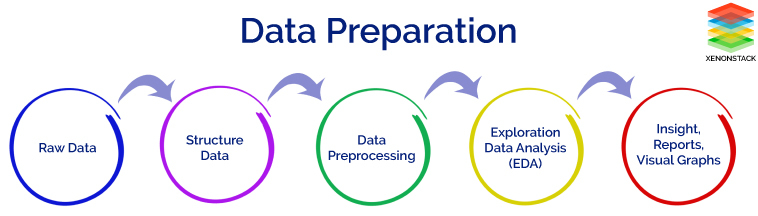## Data Pre-processing and Feature Engineering

We spend a lot of time refining our raw data. Data pre-processing and Feature Engineering plays a key role in any data process

Data Pre-processing refers to  Data Integration, Data Analysis, Data cleaning, Data Transformation, and Dimension Reduction

Data preprocessing is the process of cleaning and preparing the raw data to enable feature engineering

Feature Engineering covers various data engineering techniques such as adding/removing relevant features, handling missing data, encoding the data, handling categorical variables, etc

Feature Engineering is one of the most crucial tasks and plays a major role in determining the outcome of a model

Feature engineering involves the creation of features, whereas preprocessing involves cleaning the data.

The Data pre-processing, Feature Engineering, and EDA steps will be carried out in this article using Python.

## Import Python Libraries

The first step involved in ML using python is understanding and playing around with our data using libraries. Here is the link to the dataset.

Import all libraries which are required for our analysis, such as Data Loading, Statistical analysis, Visualizations, Data Transformations, Merge and Joins, etc.

Pandas and Numpy have been used for Data Manipulation and numerical Calculations

Matplotlib and Seaborn have been used for Data visualizations.

```import pandas as pd
import numpy as np
import matplotlib.pyplot as plt
import seaborn as sns
#to ignore warnings
import warnings
warnings.filterwarnings('ignore')```

The Pandas library offers a wide range of possibilities for loading data into the pandas DataFrame from files like JSON, .csv, .xlsx, .sql, .pickle, .html, .txt, images etc.

Most of the data are available in a tabular format of CSV files. It is trendy and easy to access. Using the read_csv() function, data can be converted to a pandas DataFrame.

In this article, the data to predict Used car price is being used as an example. In this dataset, we are trying to analyze the used car’s price and how EDA focuses on identifying the factors influencing the car price. We have stored the data in the DataFrame data.

`data = pd.read_csv("used_cars.csv")`

Analyzing the data

Before we make any inferences, we listen to our data by examining all variables in the data.

The main goal of data understanding is to gain general insights about the data, which covers the number of rows and columns, values in the data, datatypes, and Missing values in the dataset.

shape – shape will display the number of observations(rows) and features(columns) in the dataset

There are 7253 observations and 14 variables in our dataset

head()  will display the top 5 observations of the dataset

`data.head()`

tail() will display the last 5 observations of the dataset

`data.tail()`

info() helps to understand the data type and information about data, including the number of records in each column, data having null or not null, Data type, the memory usage of the dataset

`data.info()`

data.info() shows the variables Mileage, Engine, Power, Seats, New_Price, and Price have missing values. Numeric variables like Mileage, Power are of datatype as  float64 and int64. Categorical variables like Location, Fuel_Type, Transmission, and Owner Type are of object data type

#### Check for duplication

nunique() based on several unique values in each column and the data description, we can identify the continuous and categorical columns in the data. Duplicated data can be handled or removed based on further analysis

`data.nunique()`

#### Missing values Calculation

isnull() is widely been in all pre-processing steps to identify null values in the data

In our example, data.isnull().sum() is used to get the number of missing records in each column

`data.isnull().sum()`

The below code helps to calculate the percentage of missing values in each column

`(data.isnull().sum()/(len(data)))*100`

The percentage of missing values for the columns New_Price and Price is ~86% and ~17%, respectively.

## Data Reduction

Some columns or variables can be dropped if they do not add value to our analysis.

In our dataset, the column S.No have only ID values, assuming they don’t have any predictive power to predict the dependent variable.

```# Remove S.No. column from data
data = data.drop(['S.No.'], axis = 1)
data.info()```

We start our Feature Engineering as we need to add some columns required for analysis.

## Feature Engineering

Feature engineering refers to the process of using domain knowledge to select and transform the most relevant variables from raw data when creating a predictive model using machine learning or statistical modeling. The main goal of Feature engineering is to create meaningful data from raw data.

## Creating Features

We will play around with the variables Year and Name in our dataset. If we see the sample data, the column “Year” shows the manufacturing year of the car.

It would be difficult to find the car’s age if it is in year format as the Age of the car is a contributing factor to Car Price.

Introducing a new column, “Car_Age” to know the age of the car

```from datetime import date
date.today().year
data['Car_Age']=date.today().year-data['Year']

Since car names will not be great predictors of the price in our current data. But we can process this column to extract important information using brand and Model names. Let’s split the name and introduce new variables “Brand” and “Model”

`data['Brand'] = data.Name.str.split().str.get(0)`
`data['Model'] = data.Name.str.split().str.get(1) + data.Name.str.split().str.get(2)`
`data[['Name','Brand','Model']]`

## Data Cleaning/Wrangling

Some names of the variables are not relevant and not easy to understand. Some data may have data entry errors, and some variables may need data type conversion. We need to fix this issue in the data.

In the example, The brand name ‘Isuzu’ ‘ISUZU’ and ‘Mini’ and ‘Land’ looks incorrect. This needs to be corrected

```print(data.Brand.unique())
print(data.Brand.nunique())```
```searchfor = ['Isuzu' ,'ISUZU','Mini','Land']
`data["Brand"].replace({"ISUZU": "Isuzu", "Mini": "Mini Cooper","Land":"Land Rover"}, inplace=True)`

We have done the fundamental data analysis, Featuring, and data clean-up. Let’s move to the EDA process

Voila!! Our Data is ready to perform EDA.

## EDA Exploratory Data Analysis

Exploratory Data Analysis refers to the crucial process of performing initial investigations on data to discover patterns to check assumptions with the help of summary statistics and graphical representations.

• EDA can be leveraged to check for outliers, patterns, and trends in the given data.
• EDA helps to find meaningful patterns in data.
• EDA provides in-depth insights into the data sets to solve our business problems.
• EDA gives a clue to impute missing values in the dataset

## Statistics Summary

The information gives a quick and simple description of the data.

Can include Count, Mean, Standard Deviation, median, mode, minimum value, maximum value, range, standard deviation, etc.

Statistics summary gives a high-level idea to identify whether the data has any outliers, data entry error, distribution of data such as the data is normally distributed or left/right skewed

In python, this can be achieved using describe()

describe() function gives all statistics summary of data

describe()– Provide a statistics summary of data belonging to numerical datatype such as int, float

`data.describe().T`
`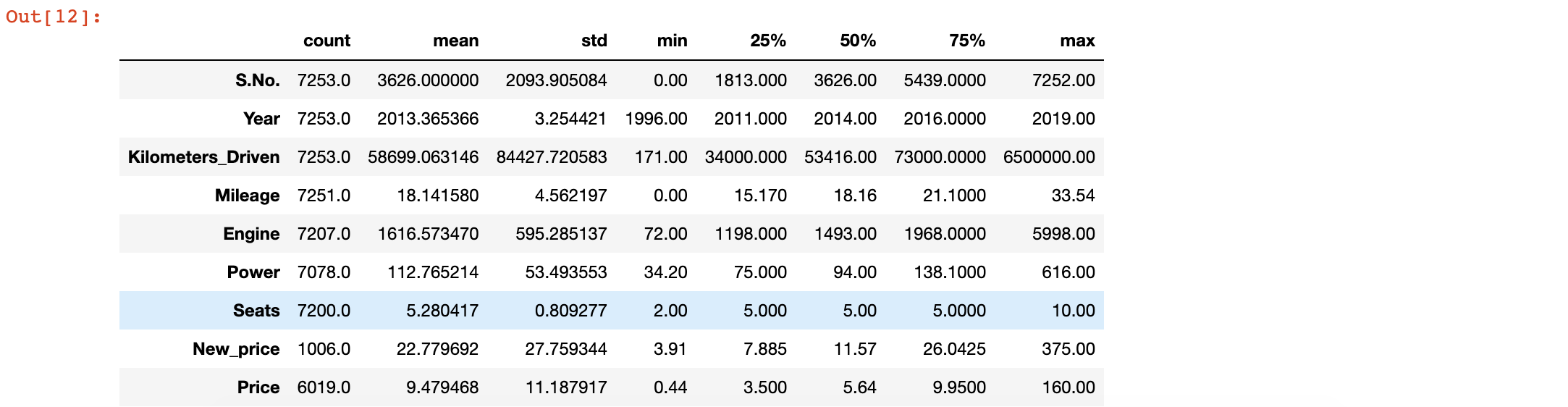`

From the statistics summary, we can infer the below findings :

• Years range from 1996- 2019 and has a high in a range which shows used cars contain both latest models and old model cars.
• On average of Kilometers-driven in Used cars are ~58k KM. The range shows a huge difference between min and max as max values show 650000 KM shows the evidence of an outlier. This record can be removed.
• Min value of Mileage shows 0 cars won’t be sold with 0 mileage. This sounds like a data entry issue.
• It looks like Engine and Power have outliers, and the data is right-skewed.
• The average number of seats in a car is 5. car seat is an important feature in price contribution.
• The max price of a used car is 160k which is quite weird, such a high price for used cars. There may be an outlier or data entry issue.

describe(include=’all’) provides a statistics summary of all data, include object, category etc

`data.describe(include='all').T`
`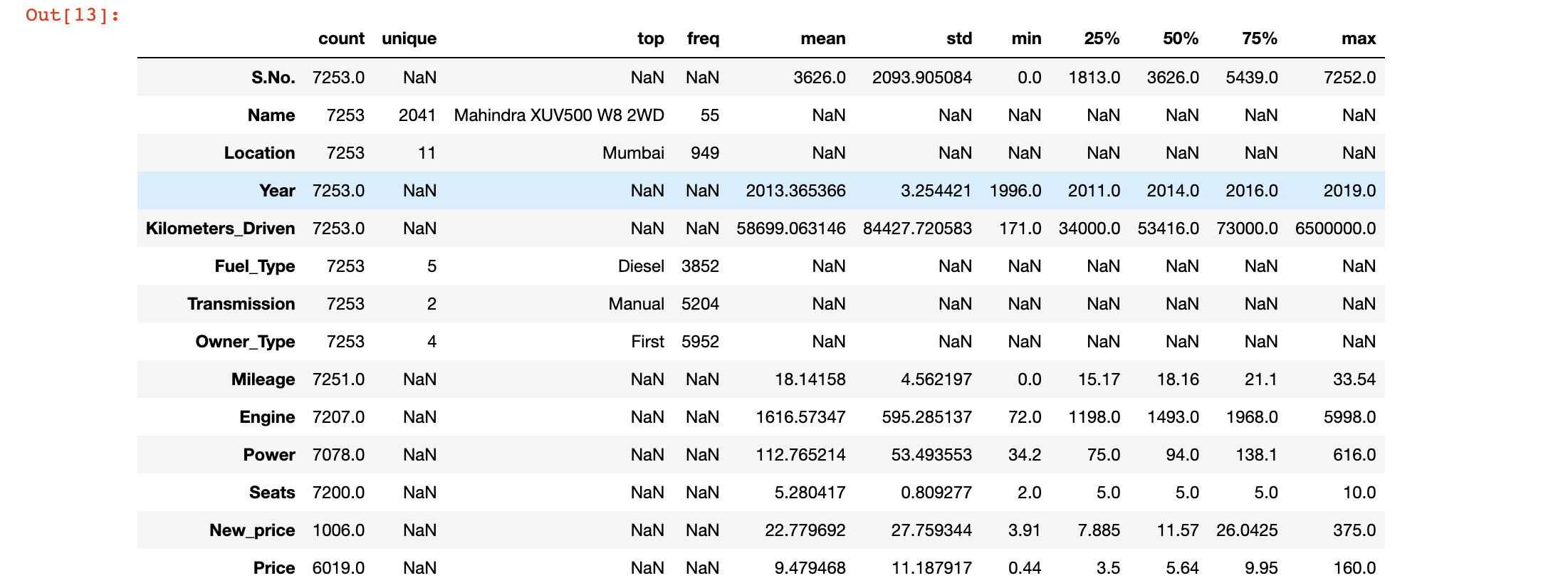`

Before we do EDA, lets separate Numerical and categorical variables for easy analysis

```cat_cols=data.select_dtypes(include=['object']).columns
num_cols = data.select_dtypes(include=np.number).columns.tolist()
print("Categorical Variables:")
print(cat_cols)
print("Numerical Variables:")
print(num_cols)```

## EDA Univariate Analysis

Analyzing/visualizing the dataset by taking one variable at a time:

Data visualization is essential; we must decide what charts to plot to better understand the data. In this article, we visualize our data using Matplotlib and Seaborn libraries.

Matplotlib is a Python 2D plotting library used to draw basic charts we use Matplotlib.

Seaborn is also a python library built on top of Matplotlib that uses short lines of code to create and style statistical plots from Pandas and Numpy

Univariate analysis can be done for both Categorical and Numerical variables.

Categorical variables can be visualized using a Count plot, Bar Chart, Pie Plot, etc.

Numerical Variables can be visualized using Histogram, Box Plot, Density Plot, etc.

In our example, we have done a Univariate analysis using Histogram and  Box Plot for continuous Variables.

In the below fig, a histogram and box plot is used to show the pattern of the variables, as some variables have skewness and outliers.

```for col in num_cols:
print(col)
print('Skew :', round(data[col].skew(), 2))
plt.figure(figsize = (15, 4))
plt.subplot(1, 2, 1)
data[col].hist(grid=False)
plt.ylabel('count')
plt.subplot(1, 2, 2)
sns.boxplot(x=data[col])
plt.show()```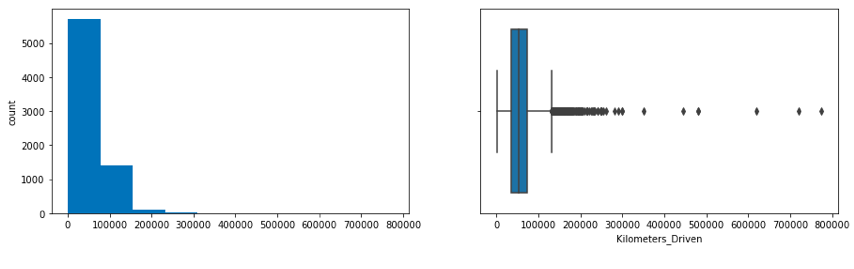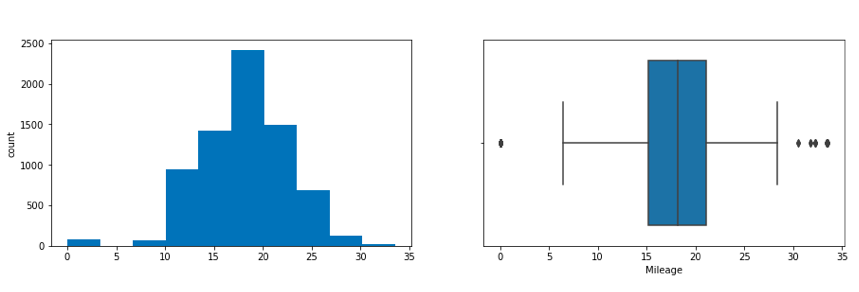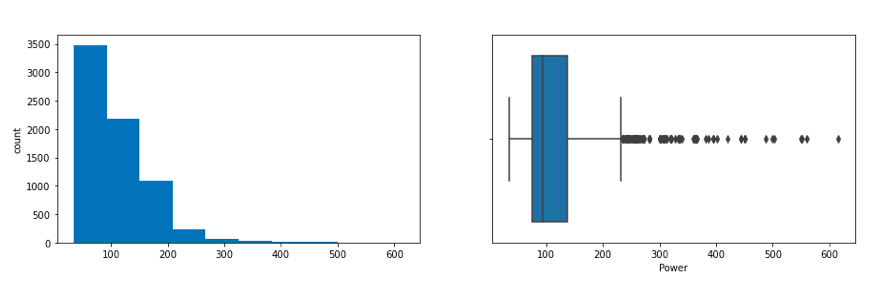Price and Kilometers Driven are right skewed for this data to be transformed, and all outliers will be handled during imputation

categorical variables are being visualized using a count plot. Categorical variables provide the pattern of factors influencing car price

```fig, axes = plt.subplots(3, 2, figsize = (18, 18))
fig.suptitle('Bar plot for all categorical variables in the dataset')
sns.countplot(ax = axes[0, 0], x = 'Fuel_Type', data = data, color = 'blue',
order = data['Fuel_Type'].value_counts().index);
sns.countplot(ax = axes[0, 1], x = 'Transmission', data = data, color = 'blue',
order = data['Transmission'].value_counts().index);
sns.countplot(ax = axes[1, 0], x = 'Owner_Type', data = data, color = 'blue',
order = data['Owner_Type'].value_counts().index);
sns.countplot(ax = axes[1, 1], x = 'Location', data = data, color = 'blue',
order = data['Location'].value_counts().index);
sns.countplot(ax = axes[2, 0], x = 'Brand', data = data, color = 'blue',
sns.countplot(ax = axes[2, 1], x = 'Model', data = data, color = 'blue',
axes.tick_params(labelrotation=45);
axes.tick_params(labelrotation=90);
axes.tick_params(labelrotation=90);```

From the count plot, we can have below observations

• Mumbai has the highest number of cars available for purchase, followed by Hyderabad and Coimbatore
• ~53% of cars have fuel type as Diesel this shows diesel cars provide higher performance
• ~72% of cars have manual transmission
• ~82 % of cars are First owned cars. This shows most of the buyers prefer to purchase first-owner cars
• ~20% of cars belong to the brand Maruti followed by 19% of cars belonging to Hyundai
• WagonR ranks first among all models which are available for purchase

## Data Transformation

Before we proceed to Bi-variate Analysis, Univariate analysis demonstrated the data pattern as some variables to be transformed.

Price and Kilometer-Driven variables are highly skewed and on a larger scale. Let’s do log transformation.

Log transformation can help in normalization, so this variable can maintain standard scale with other variables:

```# Function for log transformation of the column
def log_transform(data,col):
for colname in col:
if (data[colname] == 1.0).all():
data[colname + '_log'] = np.log(data[colname]+1)
else:
data[colname + '_log'] = np.log(data[colname])
data.info()```
`log_transform(data,['Kilometers_Driven','Price'])`
```#Log transformation of the feature 'Kilometers_Driven'
sns.distplot(data["Kilometers_Driven_log"], axlabel="Kilometers_Driven_log");```

## EDA Bivariate Analysis

Now, let’s move ahead with bivariate analysis. Bivariate Analysis helps to understand how variables are related to each other and the relationship between dependent and independent variables present in the dataset.

For Numerical variables, Pair plots and Scatter plots are widely been used to do Bivariate Analysis.

A Stacked bar chart can be used for categorical variables if the output variable is a classifier. Bar plots can be used if the output variable is continuous

In our example, a pair plot has been used to show the relationship between two Categorical variables.

```plt.figure(figsize=(13,17))
sns.pairplot(data=data.drop(['Kilometers_Driven','Price'],axis=1))
plt.show()```

Pair Plot provides below insights:

• The variable Year has a positive correlation with price and mileage
• A year has a Negative correlation with kilometers-Driven
• Mileage is negatively correlated with Power
• As power increases, mileage decreases
• Car with recent make is higher at prices. As the age of the car increases price decreases
• Engine and Power increase, and the price of the car increases

A bar plot can be used to show the relationship between Categorical variables and continuous variables

```fig, axarr = plt.subplots(4, 2, figsize=(12, 18))
data.groupby('Location')['Price_log'].mean().sort_values(ascending=False).plot.bar(ax=axarr, fontsize=12)
axarr.set_title("Location Vs Price", fontsize=18)
data.groupby('Transmission')['Price_log'].mean().sort_values(ascending=False).plot.bar(ax=axarr, fontsize=12)
axarr.set_title("Transmission Vs Price", fontsize=18)
data.groupby('Fuel_Type')['Price_log'].mean().sort_values(ascending=False).plot.bar(ax=axarr, fontsize=12)
axarr.set_title("Fuel_Type Vs Price", fontsize=18)
data.groupby('Owner_Type')['Price_log'].mean().sort_values(ascending=False).plot.bar(ax=axarr, fontsize=12)
axarr.set_title("Owner_Type Vs Price", fontsize=18)
axarr.set_title("Brand Vs Price", fontsize=18)
axarr.set_title("Model Vs Price", fontsize=18)
data.groupby('Seats')['Price_log'].mean().sort_values(ascending=False).plot.bar(ax=axarr, fontsize=12)
axarr.set_title("Seats Vs Price", fontsize=18)
data.groupby('Car_Age')['Price_log'].mean().sort_values(ascending=False).plot.bar(ax=axarr, fontsize=12)
axarr.set_title("Car_Age Vs Price", fontsize=18)
sns.despine()```

Observations

• The price of cars is high in Coimbatore and less price in Kolkata and Jaipur
• Automatic cars have more price than manual cars.
• Diesel and Electric cars have almost the same price, which is maximum, and LPG cars have the lowest price
• First-owner cars are higher in price, followed by a second
• The third owner’s price is lesser than the Fourth and above
• Lamborghini brand is the highest in price
• Gallardocoupe Model is the highest in price
• 2 Seater has the highest price followed by 7 Seater
• The latest model cars are high in price

## EDA Multivariate Analysis

As the name suggests, Multivariate analysis looks at more than two variables. Multivariate analysis is one of the most useful methods to determine relationships and analyze patterns for any dataset.

A heat map is widely been used for Multivariate Analysis

Heat Map gives the correlation between the variables, whether it has a positive or negative correlation.

In our example heat map shows the correlation between the variables.

```plt.figure(figsize=(12, 7))
sns.heatmap(data.drop(['Kilometers_Driven','Price'],axis=1).corr(), annot = True, vmin = -1, vmax = 1)
plt.show()```

From the Heat map, we can infer the following:

• The engine has a strong positive correlation to Power 0.86
• Price has a positive correlation to Engine 0.69 as well Power 0.77
• Mileage has correlated to Engine, Power, and Price negatively
• Price is moderately positive in correlation to year.
• Kilometer driven has a negative correlation to year not much impact on the price
• Car age has a negative correlation with Price
• car Age is positively correlated to Kilometers-Driven as the Age of the car increases; then the kilometer will also increase of car has a negative correlation with Mileage this makes sense

## Impute Missing values

Missing data arise in almost all statistical analyses. There are many ways to impute missing values; we can impute the missing values by their Mean, median, most frequent, or zero values and use advanced imputation algorithms like KNNRegularization, etc.

We cannot impute the data with a simple Mean/Median. We must need business knowledge or common insights about the data. If we have domain knowledge, it will add value to the imputation. Some data can be imputed on assumptions.

In our dataset, we have found there are missing values for many columns like Mileage, Power, and Seats.

We observed earlier some observations have zero Mileage. This looks like a data entry issue. We could fix this by filling null values with zero and then the mean value of Mileage since Mean and Median values are nearly the same for this variable chosen Mean to impute the values.

```data.loc[data["Mileage"]==0.0,'Mileage']=np.nan
data.Mileage.isnull().sum()```
`data['Mileage'].fillna(value=np.mean(data['Mileage']),inplace=True)`

Similarly, imputation for Seats. As we mentioned earlier, we need to know common insights about the data.

Let’s assume some cars brand and Models have features like Engine, Mileage, Power, and Number of seats that are nearly the same. Let’s impute those missing values with the existing data:

```data.Seats.isnull().sum()
data['Seats'].fillna(value=np.nan,inplace=True)
data['Seats']=data.groupby(['Model','Brand'])['Seats'].apply(lambda x:x.fillna(x.median()))
data['Engine']=data.groupby(['Brand','Model'])['Engine'].apply(lambda x:x.fillna(x.median()))
data['Power']=data.groupby(['Brand','Model'])['Power'].apply(lambda x:x.fillna(x.median()))```

In general, there are no defined or perfect rules for imputing missing values in a dataset. Each method can perform better for some datasets but may perform even worse. Only practice and experiments give the knowledge which works better.

## Conclusion

In this article, we tried to analyze the factors influencing the used car’s price.

• Data Analysis helps to find the basic structure of the dataset.
• Dropped columns that are not adding value to our analysis.
• Performed Feature Engineering by adding some columns which contribute to our analysis.
• Data Transformations have been used to normalize the columns.
• We used different visualizations for EDA like Univariate, Bi-Variate, and Multivariate Analysis.

Through EDA, we got useful insights, and below are the factors influencing the price of the car and a few takeaways

• Most of the customers prefer 2 Seat cars hence the price of the 2-seat cars is higher than other cars.
• The price of the car decreases as the Age of the car increases.
• Customers prefer to purchase the First owner rather than the Second or Third.
• Due to increased Fuel price, the customer prefers to purchase an Electric vehicle.
• Automatic Transmission is easier than Manual.

This way, we perform EDA on the datasets to explore the data and extract all possible insights, which can help in model building and better decision making.

However, this was only an overview of how EDA works; you can go deeper into it and attempt the stages on larger datasets.

If the EDA process is clear and precise, our model will work better and gives higher accuracy!